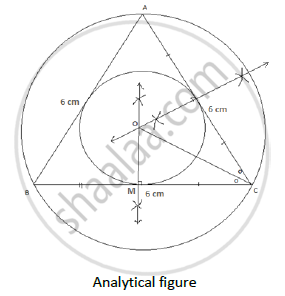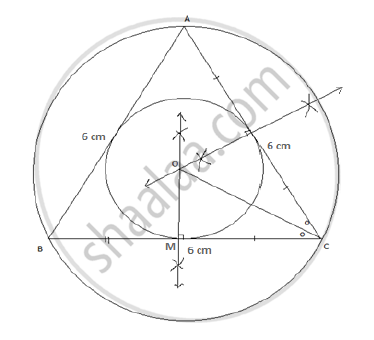# Construct the Circumcircle and Incircle of an Equilateral Triangle Abc with Side 6 Cm and Centre O. Find the Ratio of Radii of Circumcircle and Incircle. - Geometry

Construct the circumcircle and incircle of an equilateral triangle ABC with side 6 cm and centre O. Find the ratio of radii of circumcircle and incircle.

#### Solution

Construction:-Steps of Construction:-

1. Construct an equilateral triangle Δ ABC with side 6 cm.
2. Draw perpendicular bisectors of any two sides. Let O be the point of intersection.
3. Draw a circle with centre O and radius OA or OB or OC. This gives us circumcircle equilateral triangle Δ ABC.
4. Draw the bisector of ∠C . It passes through centre of the circle O.
5. Draw a circle with radius OM. This gives us incircle of the equilateral triangle Δ ABC.In an equilateral triangle angle bisector and median are same, also circumcentre and incentre are same. For equilateral triangle circumcentre divides the median in 2:1.

So the ratio of radii of circumcircle and incircle is 2:1.

Concept: Division of a Line Segment
Is there an error in this question or solution?
2013-2014 (March)

Share